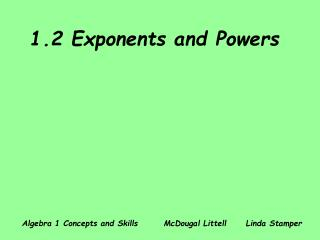DownloadDownload Presentation1.2 Exponents and Powers

# 1.2 Exponents and Powers

Download Presentation## 1.2 Exponents and Powers

- - - - - - - - - - - - - - - - - - - - - - - - - - - E N D - - - - - - - - - - - - - - - - - - - - - - - - - - -
##### Presentation Transcript

1. 1.2 Exponents and Powers Algebra 1 Concepts and Skills McDougal Littell Linda Stamper

2. An expression like 43 is called a power. 43 base   exponent word form: four to the third power four cubed factor form (meaning): 4 • 4 • 4 evaluated form: 64

3. Example 1Express the power in words. Then write the meaning. 1. Write problem. 82 2. Write word form. eight squared 3. Write meaning. (factored form) 8 • 8

4. Example 2Express the power in words. Then write the meaning. 1. Write problem. 53 2. Write word form. five cubed 3. Write meaning. 5 • 5 • 5

5. Example 3Express the power in words. Then write the meaning. 1. Write problem. y4 y to the fourth power 2. Write word form. 3. Write meaning. y • y • y • y

6. A power applies only to what is directly in front of it. Evaluate when x = 6. The problem. x2 Substitute. (6)2 Write factors. (6)(6) Simplify. 36 You could write in factored form and then substitute. x2 (6)(6) 36

7. When you evaluate exponential expressions, work within grouping symbols first. ( ) , [ ] ,{ } Example 4Evaluate when m = 4 and n = 3. Remember: In algebra work downward. Highlight or circle your answer. Skip one line after the answer. 1. Write problem. (m + n)2 2. Substitute. ((4) + (3))2 3. Simplify within parentheses. (7)2 49 4. Evaluate power.

8. When you evaluate exponential expressions, work within grouping symbols first. ( ) , [ ] ,{ } Example 5Evaluate when m = 4 and n = 3. (m2) + (n2) 1. Write problem. ((4)2)+ ((3)2) 2. Substitute. 3. Write factors. (4 • 4) + (3 • 3)Optional Step 16 + 9 4. Simplify within parentheses. 25 5. Simplify. When parentheses are nested work from the inside going out.

9. Example 6Evaluate when a = 5. Remember a power applies only to what is directly in front of it! 1. Write problem. 2a2 2. Substitute. 2(5)2 3. Evaluate power. 2(25) 50 4. Simplify.

10. Example 7Evaluate when a = 5. 1. Write problem. (2a)2 2. Substitute. (2(5))2 3. Simplify within parentheses. (10)2 100 4. Evaluate power.

11. Example 8A box has the shape of a cube. Each edge s is 8 inches long. Find the volume in cubic inches. 1. Write formula. V = s3 2. Substitute. = (8)3 3. Write factors. Optional Step = 8 • 8 • 8 4. Simplify. = 512 5. Write a sentence. The volume of the box is 512 cubic inches. This cannot be written as 5123inches!

12. Homework 1-A2 Pages 12-14, # 1-57